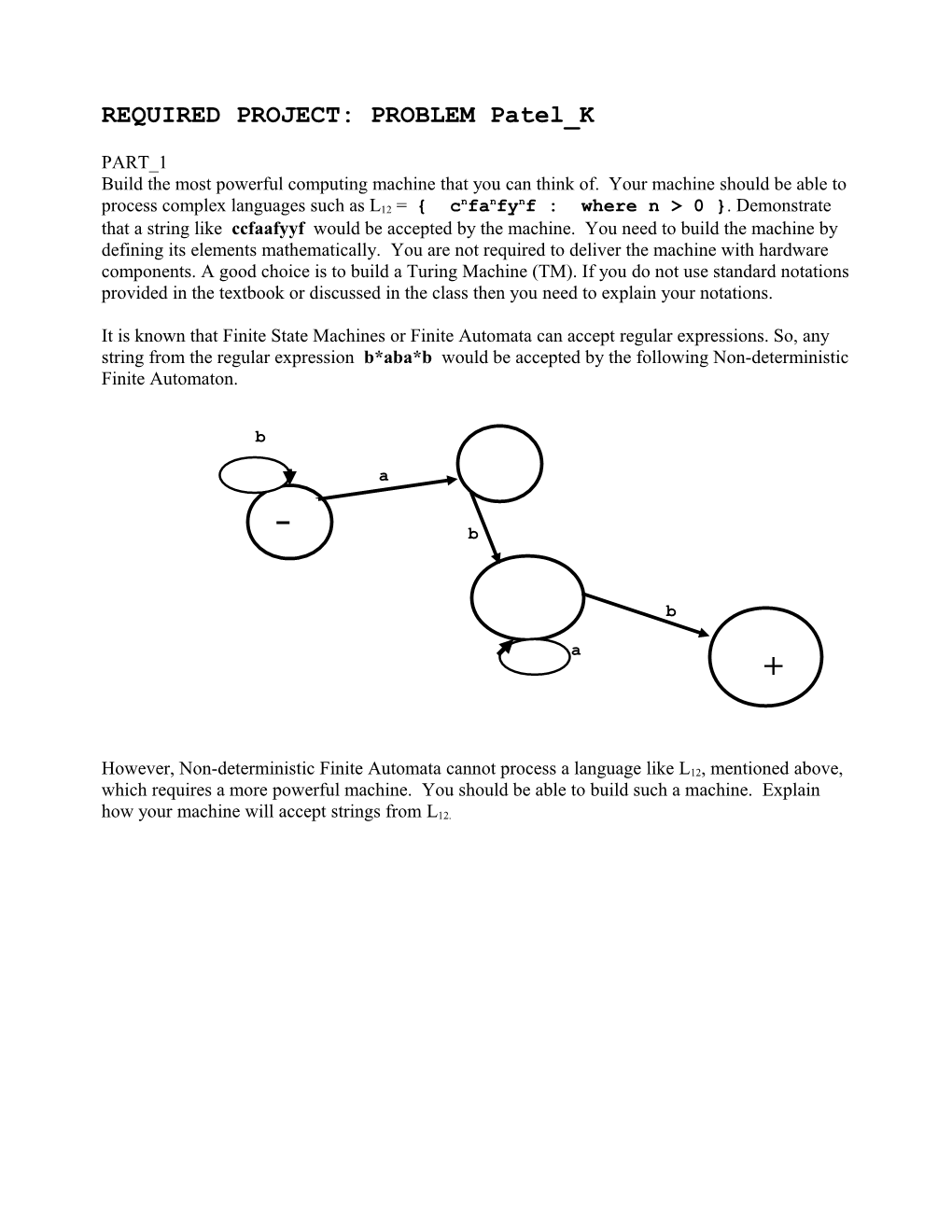# REQUIRED PROJECT:PROBLEM Patel KREQUIRED PROJECT:PROBLEM Patel_K

PART_1

Build the most powerful computing machine that you can think of. Your machine should be able to process complex languages such as L12 = { cnfanfynf : where n > 0 }. Demonstrate that a string like ccfaafyyf would be accepted by the machine. You need to build the machine by defining its elements mathematically. You are not required to deliver the machine with hardware components. A good choice is to build a Turing Machine (TM). If you do not use standard notations provided in the textbook or discussed in the class then you need to explain your notations.

It is known that Finite State Machines or Finite Automata can accept regular expressions. So, any string from the regular expression b*aba*b would be accepted by the following Non-deterministic Finite Automaton.

b

a

b

b

a

However,Non-deterministic Finite Automata cannot process a language like L12, mentioned above, which requires a more powerful machine. You should be able to build such a machine. Explain how your machine will accept strings fromL12.

PART_2:

A Turning Machine has a finite set of states with one START state and some (may be none) HALT states. We always mark the START state with 1 and the HALT state with 2 (when there is only one HALT state).

(b, b, R)

(b, b, R)

(a, a, R) (a, a, R) (Δ, Δ, R)

Figure 1. A Turing Machine for { b*ab*a }

The Turing Machine given above accepts the language: { b*ab*a }. When an input is given it is processed by the transitions as the Turing Machine goes from state to state starting from the start state. The input is accepted by reaching the HALT state. If the HALT state and its incoming transition are destroyed then no input is accepted. The Turing Machine of Figure 1 would look like the machine given below after the destruction of the HALT state and its incoming transition.

(b, b, R)

(b, b, R)

(a, a, R) (a, a, R)

Figure 2. The Turing Machine of Figure 1 after the HALT state and its incoming transition are destroyed.

If the input is: aba, then the machine will start in the START state and reach state 4 after going through the loop of state 3. However, the input is not accepted.